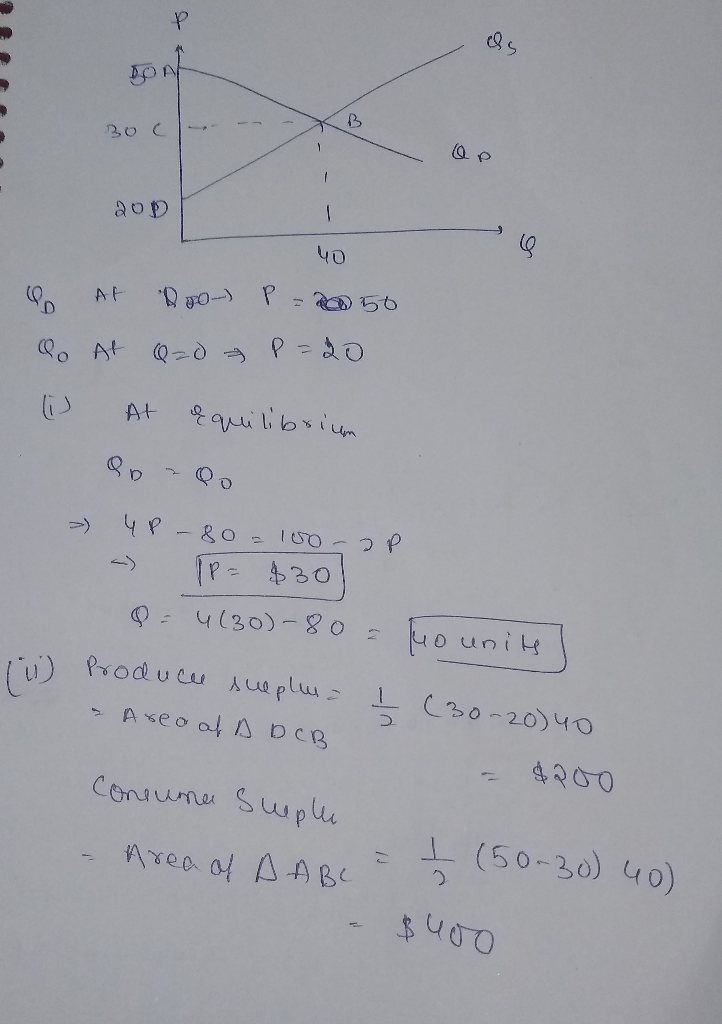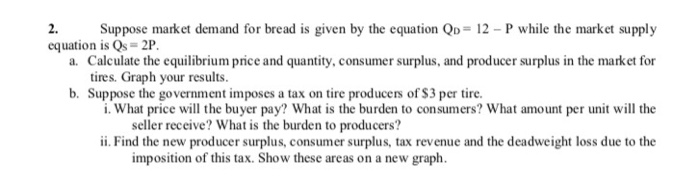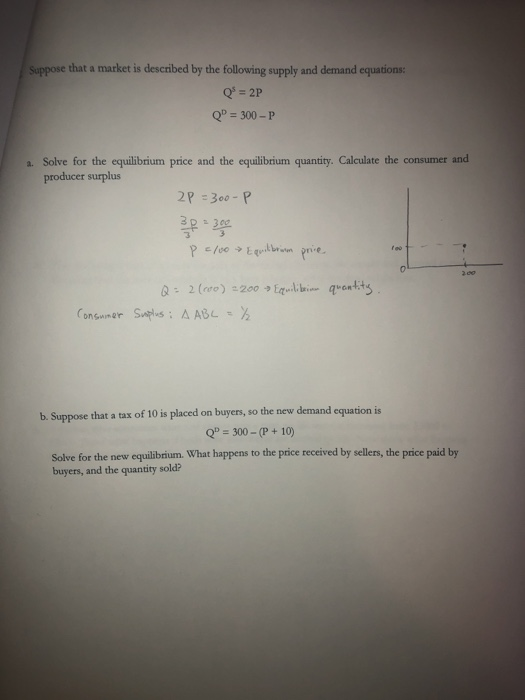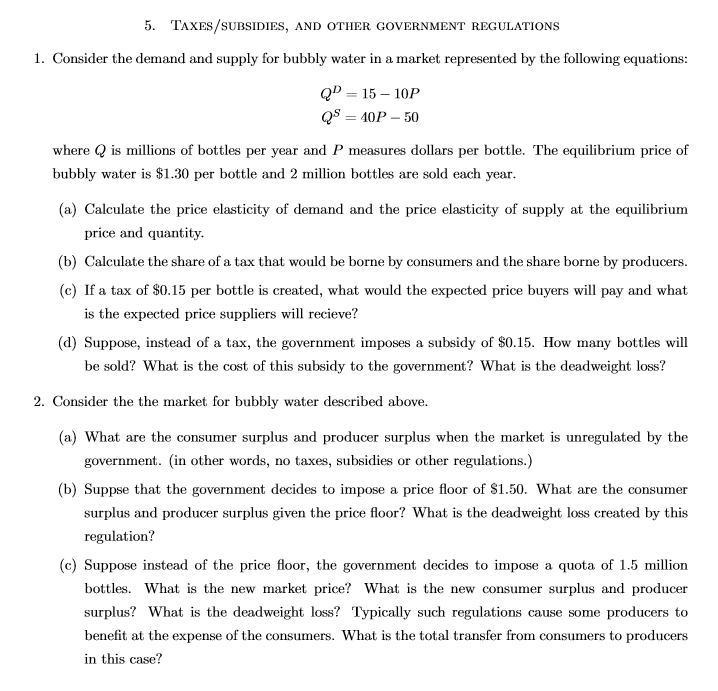Question

Supply and demand in the regional vegetable market can be described with the following equations:

Qo = 4P - 80
Qd = 100 - 2P

The amount (Q) is expressed in quintals and the P in dollars per quintal.

to. What are the equilibrium conditions in the vegetable market?

b. Calculate the consumer surplus, the producer surplus and the total surplus in the vegetable market.

c. Which economic sector (consumers or producers) would have the greatest weight if the government prohibited the consumption of vegetables in the population?C)since it is observed that the vegetable market is relatively inelastic in nature as vegetable is a necessity if a consumption of vegetables is seen then the consumers should be more affected.

#### Earn Coins

Coins can be redeemed for fabulous gifts.

Similar Homework Help Questions
• ### The supply and demand for broccoli are described by the following equations:

The supply and demand for broccoli are described by the following equations:Qs=4P-80 Qd=100-2Pwhere Q is the quantity of broccoli and P is its price.(a) Graph the supply curve and the demand curve.(b) What is equilibrium price and quantity?(c) Calculate consumer surplus, producer surplus, and total surplus at the equilibrium. Indicate consumer surplus and producer surplus on your diagram.(d) Suppose a dictator who hated broccoli was to tax the sellers of the vegetable. Explain what determines who bears the largest burden -...

• ### The following equations represent the inverse supply and demand functions in the market for Good A:...

The following equations represent the inverse supply and demand functions in the market for Good A: PC =80-1⁄2QD PP =14+QS where PC and PP are the prices paid by consumers and received by producers respectively. QD and QS are the quantities demanded and supplied, respectively. Suppose the government is considering imposing a tax of \$6 per unit of Good A. a) Compute the competitive market equilibrium price and output without the tax. b) Compute producer surplus and consumer surplus without...

• ### The following equations represent the inverse supply and demand functions in the market for Good A:...

The following equations represent the inverse supply and demand functions in the market for Good A: PC = 80 - ½ QD PP = 14 + QS where PC and PP are the prices paid by consumers and received by producers respectively. QD and QS are the quantities demanded and supplied, respectively. c) (2pts) Compute the competitive market equilibrium price and output with the tax. d) (4pts) Compute producer surplus and consumer surplus with the tax.e the government is considering...

• ### The following equations represent the inverse supply and demand functions in the market for Good A:...

The following equations represent the inverse supply and demand functions in the market for Good A: PC = 80 - ½ QD PP = 14 + QS where PC and PP are the prices paid by consumers and received by producers respectively. QD and QS are the quantities demanded and supplied, respectively. Suppose the government is considering imposing a tax of \$6 per unit of Good A. a) (2pts) Compute the competitive market equilibrium price and output without the tax....

• ### 2. (Total: 15 pts) The following equations represent the inverse supply and demand functions in the market for Good A:...

2. (Total: 15 pts) The following equations represent the inverse supply and demand functions in the market for Good A: PC = 80 - ½ QD PP = 14 + QS where PC and PP are the prices paid by consumers and received by producers respectively. QD and QS are the quantities demanded and supplied, respectively. Suppose the government is considering imposing a tax of \$6 per unit of Good A.   a) (2pts) Compute the competitive market equilibrium price and...

• ### The demand and supply conditions of market for beer are given by the following equations: Qd...The demand and supply conditions of market for beer are given by the following equations: Qd = 72 - P and Qs = -18 + P a) Find the initial equilibrium price and quantity. b) Calculate the consumer surplus and producer surplus for the equilibrium. c) Suppose that government impose a price floor at P=66 to control the consumption of beer. Is this policy effective? What are price and quantity consumed after this intervention of government? d) Going back to...

• ### Suppose that the market demand and supply equations in a perfectly competitive market are QD =...

Suppose that the market demand and supply equations in a perfectly competitive market are QD = 16 − 4P and QS = −2 + 2P, respectively. What is the full economic price if the government imposes a price ceiling of \$2?

• ### Suppose market demand for bread is given by the equation QD = 12-P while the market...Suppose market demand for bread is given by the equation QD = 12-P while the market supply equation is Qs = 2P. a. Calculate the equilibrium price and quantity, consumer surplus, and producer surplus in the market for tires. Graph your results. b. Suppose the government imposes a tax on tire producers of \$3 per tire. i. What price will the buyer pay? What is the burden to consumers? What amount per unit will the seller receive? What is the...

• ### part b Suppose that a market is described by the following supply and demand equations Q...part b Suppose that a market is described by the following supply and demand equations Q = 2P QP = 300-P a. Solve for the equilibrium price and the equilibrium quantity. Calculate the consumer and producer surplus 2P =300-P P 2100 Equilibrium price quantity Q = 2(000) =200 Equilibri Suplus: A ABL = ²2 Consumer b. Suppose that a tax of 10 is placed on buyers, so the new demand equation is Q" = 300 - (P+10) Solve for the...

• ### 5. TAXES/SUBSIDIES, AND OTHER GOVERNMENT REGULATIONS 1. Consider the demand and supply for bubbly water in...5. TAXES/SUBSIDIES, AND OTHER GOVERNMENT REGULATIONS 1. Consider the demand and supply for bubbly water in a market represented by the following equations: QD = 15 - 10P QS = 40P - 50 where Q is millions of bottles per year and P measures dollars per bottle. The equilibrium price of bubbly water is \$1.30 per bottle and 2 million bottles are sold each year. (a) Calculate the price elasticity of demand and the price elasticity of supply at the...